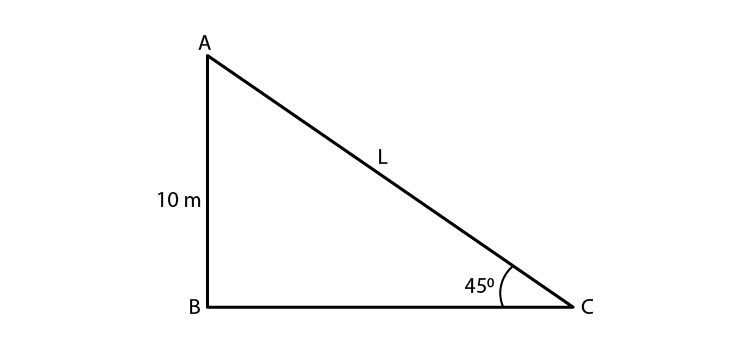# An electric pole is 10 m high. A steel wire tied to top of the pole is affixed at a point on the ground to keep the pole up right. If the wire makes an angle of 45° with the horizontal through the foot of the pole, find the length of the wire.Given,

Height of the electric pole = 10 m = AB

The angle made by steel wire with ground (horizontal) θ = 45°

Let length of wire = L = AC

Hence, from the figure formed we have ABC as a right triangle.

Sin θ = opposite side/hypotenuse

Sin 45° = AB/AC

1/√2 = 10m/L

L = 10√2 m

Therefore, the length of the wire is 10√2 m(1)(0)